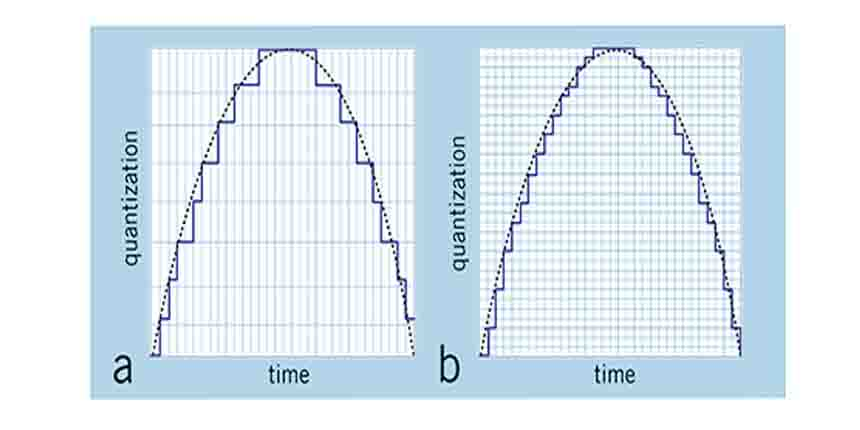# bit rate bit depth by pratik

May 05, 2018 Written by Pratik srivastava Bit rate bit depthBit rate Bit depth

1 Bitrate, as the name implies, describes the rate at which bits are transferred from one location to another. In other words, it measures how much data is transmitted in a given amount of time

2. Bitrate is commonly measured in bits per second (bps), kilobits per second (Kbps), or megabits per second (Mbps). For example, a DSL connection may be able to download data at 768 kbps, while a Firewire 800 connection can transfer data up to 800 Mbps.

3.In networking and digital telecommunications, bit rate refers to the per-second measurement of data that passes through a communications network. In this context, bit rate is synonymous with data transfer rate (DTR).

4.For multimedia encoding, bit rate refers to the number of bits used per unit of playback time, such as video or audio after compression (encoding). Multimedia size and output quality often depend on the bit rate used during encoding.

5.A video bitrate is the number of bits that are processed in a unit of time. Remember that 1 byte consists of 8 bits. Video data rates are given in bits per second. The data rate for a video file is the bitrate. So a data rate specification for video content that runs at 1 megabyte per second would be given as a bitrate of 8 megabits per second (8 mbps)

6.The bitrate for an HD Blu-ray video is typically in the range of 20 mbps, standard-definition DVD is usually 6 mbps, high-quality web video often runs at about 2 mbps, and video for phones is typically given in the kilobits (kbps). For example, these are the targets we usually see for H.264 streaming:

7. In digital audio , bit depth describes the potential accuracy of a particular piece of hardware or software that processes audio data. In general, the more bits that are available, the more accurate the resulting output from the data being processed.

8.The sample size—more accurately, the number of bits used to describe each sample—is called the bit depth or word length. The number of bits transmitted per second is the bit rate. Let's take a look at this as it applies to digital audio.

9.Color depth or colour depth (see spelling differences), also known as bit depth, is either the number of bits used to indicate the color of a single pixel, in a bitmappedimage or video frame buffer, or the number of bits used for each color component of a single pixel.

10. Bit depth is the number of bit s you have in which to describe something. Each additional bit in a binary number doubles the number of possibilities. By the time you have a 16-bit sequence, there are 65,536 possible levels.